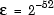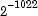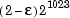# Variables in Matlab

Posted by SUYOG PATIL on 7:50 PM in , , ,
Variables
Like most other programming languages, the MATLAB language provides
mathematical expressions, but unlike most programming languages, these
expressions involve entire matrices.
MATLAB does not require any type declarations or dimension statements.
When MATLAB encounters a new variable name, it automatically creates the
variable and allocates the appropriate amount of storage. If the variable
already exists, MATLAB changes its contents and, if necessary, allocates
new storage. For example,
num_students = 25
creates a 1-by-1 matrix named num_students and stores the value 25 in its
single element. To view the matrix assigned to any variable, simply enter
the variable name.
Variable names consist of a letter, followed by any number of letters, digits, or
underscores. MATLAB is case sensitive; it distinguishes between uppercase
and lowercase letters. A and a are not the same variable.
Although variable names can be of any length, MATLAB uses only the first
N characters of the name, (where N is the number returned by the function
namelengthmax), and ignores the rest. Hence, it is important to make
each variable name unique in the first N characters to enable MATLAB to
distinguish variables.
N = namelengthmax
N =
63
The genvarname function can be useful in creating variable names that are
both valid and unique.
Numbers
MATLAB uses conventional decimal notation, with an optional decimal point
and leading plus or minus sign, for numbers. Scientific notation uses the
letter e to specify a power-of-ten scale factor. Imaginary numbers use either i
or j as a suffix. Some examples of legal numbers are
3 -99 0.0001
9.6397238 1.60210e-20 6.02252e23
1i -3.14159j 3e5i
MATLAB stores all numbers internally using the long format specified by
the IEEE® floating-point standard. Floating-point numbers have a finite
precision of roughly 16 significant decimal digits and a finite range of roughly
10-308 to 10+308.
Numbers represented in the double format have a maximum precision of
52 bits. Any double requiring more bits than 52 loses some precision. For
example, the following code shows two unequal values to be equal because
they are both truncated:
x = 36028797018963968;
y = 36028797018963972;
x == y
ans =1
Integers have available precisions of 8-bit, 16-bit, 32-bit, and 64-bit. Storing
the same numbers as 64-bit integers preserves precision:
x = uint64(36028797018963968);
y = uint64(36028797018963972);
x == y
ans =0
The section “Avoiding Common Problems with Floating-Point Arithmetic”
gives a few of the examples showing how IEEE floating-point arithmetic
affects computations in MATLAB. For more examples and information, see
Technical Note 1108 — Common Problems with Floating-Point Arithmetic.
MATLAB software stores the real and imaginary parts of a complex number.
It handles the magnitude of the parts in different ways depending on the
context. For instance, the sort function sorts based on magnitude and
resolves ties by phase angle.
sort([3+4i, 4+3i])
ans =
4.0000 + 3.0000i 3.0000 + 4.0000i
This is because of the phase angle:
angle(3+4i)
ans =
0.9273
angle(4+3i)
ans =
0.6435
The “equal to” relational operator == requires both the real and imaginary
parts to be equal. The other binary relational operators > <, >=, and <= ignore
the imaginary part of the number and consider the real part only.
Operators
Expressions use familiar arithmetic operators and precedence rules.
- Subtraction
* Multiplication
/ Division
\ Left division (described in “Linear Algebra” in the
MATLAB documentation)
^ Power
' Complex conjugate transpose
( ) Specify evaluation order
Functions
MATLAB provides a large number of standard elementary mathematical
functions, including abs, sqrt, exp, and sin. Taking the square root or
logarithm of a negative number is not an error; the appropriate complex result
is produced automatically. MATLAB also provides many more advanced
mathematical functions, including Bessel and gamma functions. Most of
these functions accept complex arguments. For a list of the elementary
mathematical functions, type
help elfun
For a list of more advanced mathematical and matrix functions, type
help specfun
help elmat
Some of the functions, like sqrt and sin, are built in. Built-in functions are
part of the MATLAB core so they are very efficient, but the computational
details are not readily accessible. Other functions are implemented in the
MATLAB programing language, so their computational details are accessible.
There are some differences between built-in functions and other functions.
For example, for built-in functions, you cannot see the code. For other
functions, you can see the code and even modify it if you want.
Several special functions provide values of useful constants.
pi 3.14159265...
i Imaginary unit,
j Same as ieps Floating-point relative precision,
realmin Smallest floating-point number,
realmax Largest floating-point number,
Inf Infinity
NaN Not-a-number
Infinity is generated by dividing a nonzero value by zero, or by evaluating
well defined mathematical expressions that overflow, i.e., exceed realmax.
Not-a-number is generated by trying to evaluate expressions like 0/0 or
Inf-Inf that do not have well defined mathematical values.
The function names are not reserved. It is possible to overwrite any of them
with a new variable, such as
eps = 1.e-6realmin Smallest floating-point number,realmax Largest floating-point number,Inf-  Infinity
NaN - Not-a-number
and then use that value in subsequent calculations. The original function
can be restored with
clear eps
Examples of Expressions
You have already seen several examples of MATLAB expressions. Here are a
few more examples, and the resulting values:
rho = (1+sqrt(5))/2
rho =
1.6180
a = abs(3+4i)
a =
5
z = sqrt(besselk(4/3,rho-i))
z =
0.3730+ 0.3214i
huge = exp(log(realmax))
huge =
1.7977e+308
toobig = pi*huge
toobig =
Inf